Vectors and conics

This free course is available to start right now. Review the full course description and key learning outcomes and create an account and enrol if you want a free statement of participation.

Free course

2.6 Lines

Earlier, we found the equation of a line in the (x, y)-plane in the form ax + by = c, for some real numbers a, b and c, where a and b are not both zero. We now find an equivalent equation for a line in terms of vectors.

Let P and Q be two given points with position vectors p and q, and denote by ℓ the line that passes through P and Q. How can we find the position vector r of a point R on ℓ that lies between P and Q?

First, we use the Triangle Law for the addition of vectors to find expressions for the vectors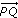and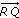; this gives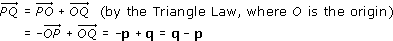and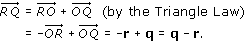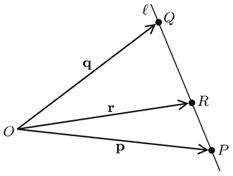Sinceandare parallel, the vector qr is parallel to the vector qp, so it must be a multiple of qp; that is,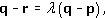Note: Two vectors are parallel if they are in the same direction or in opposite directions.

We can rearrange the above equation in the form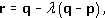so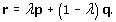This is a general formula for the position vector of a point on the line segment PQ, in the following sense: each point on ℓ between P and Q corresponds to a particular value of λ between 0 and 1, and vice versa.

In equation (2.3),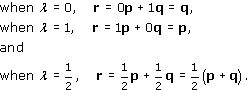This is the midpoint of the line segment PQ.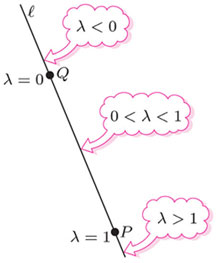Equation (2.3) also makes sense when λ > 1 and when λ < 0, and not just when λ lies between 0 and 1. In fact,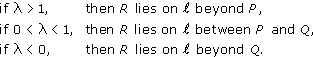Thus each point on the line ℓ has a position vector of the form of equation (2.3), for some value of λ. In other words, we can regard equation (2.3) as the vector form of the equation of the line ℓ, with λ as a parameter.

Vector form of the equation of a line

The equation of the line through the points with position vectors p and q is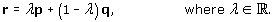Example 27

• (a) Let P and Q be the points with position vectors p = (3, 1) and q = (2, 3), respectively. Write down the vector form of the equation of the line ℓ through P and Q.

• (b) Determine the points on ℓ whose position vectors are given by equation (2.3) when λ takes the values,and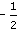.

• (c) On a single diagram, sketch P, Q, the line ℓ through P and Q, and the three points that you found in part (b).

• (a) We use equation (2.3) to obtain the vector form of the equation of ℓ as

•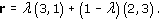• (b) Using the above formula with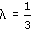,andin turn, we obtain the following position vectors:

•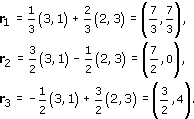• Thus the three points have Cartesian coordinates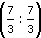,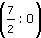and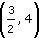, respectively.

• (c)

•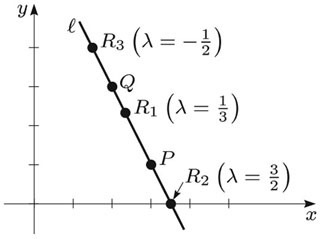The vector form of the equation of the line ℓ passing through the points (3, 1) and (2, 3) isthat is,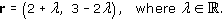We can use this equation to determine whether or not a given point lies on the line ℓ. For example, the point (5, −3) lies on ℓ if there is some real number λ such that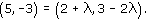Equating the corresponding components, we see that this condition reduces to a pair of simultaneous equations that must be satisfied by such a number λ: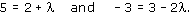These have the solution λ = 3, and hence the point (5, −3) does lie on the line ℓ.

Example 28

Let P, Q and ℓ be as in Exercise 27.

• (a) Determine the value of λ corresponding to the point (4, −1) in the vector form of the equation of ℓ: r = λ(3, 1) + (1 − λ)(2, 3).

• (b) Use the vector form of the equation of ℓ to prove that the point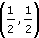does not lie on ℓ.

• (a) The vector form of the equation of ℓ is

•• Hence at the point (4, −1) on ℓ, we have

•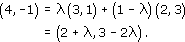• Equating corresponding components, we obtain the following pair of simultaneous equations that must be satisfied by the number λ:

•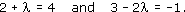• These have the solution λ = 2.

• (b) Since the vector equation of ℓ is r = λ(3, 1) + (1 − λ)(2, 3), the pointlies on ℓ if and only if there is some real number λ for which

•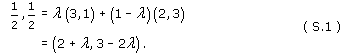• Equating corresponding components, we obtain the following pair of simultaneous equations that must be satisfied by such a number λ:

•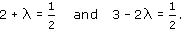• The first of these equations has solution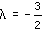, and the second has solution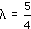.

• It follows that there is no real number λ that satisfies equation (S.1), so the pointdoes not lie on ℓ.

We can obtain another useful result from our derivation of equation (2.3).

We saw above that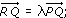it follows that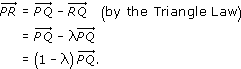Comparing these expressions forand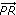, we conclude that R is the point that divides the line PQ in the ratio (1 − λ) : λ.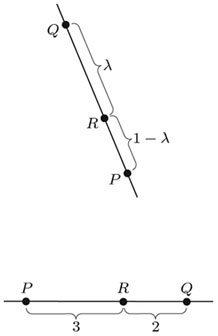For example, let PR : RQ = 3: 2. Since the ratio 3 : 2 is just the same as the ratio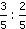, we write this equation in the form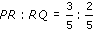, so that PR : RQ = (1 − λ) : λ with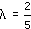. (Here we divide each expression in the ratio by the sum 3 + 2 = 5, in order to obtain the ratio in the standard form (1 − λ) : λ.)

Section Formula

The position vector r of the point that divides the line joining the points with position vectors p and q in the ratio (1 − λ) : λ is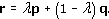Note: When q = (0, 0), r has the simple form r = λp; we shall find this fact useful later.

Example 4

Let P and Q be the points (−1, 3) and (5, 6). Determine the point R that divides the line segment PQ in the ratio 1 : 2 (thus R is one-third of the way from P to Q).

The position vectors of P and Q are p = (−1, 3) and q = (5, 6); let R have position vector r.

Since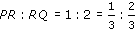, we can apply the Section Formula with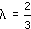; hence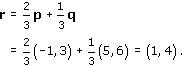Thus R is the point with coordinates (1, 4).

Example 29

Let P and Q be the points (−3, 1) and (7, −4). Determine

• (a) the point R that divides PQ in the ratio 3 : 2;

• (b) the midpoint M of PQ.

• (a) The point R divides PQ in the ratio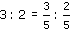. Applying the Section Formula with, we find that the position vector of R is

•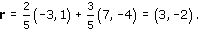• Thus R is the point (3, −2).

• (b) The midpoint M divides PQ in the ratio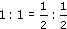. Applying the Section Formula with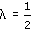, we find that the position vector of M is

•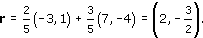• Thus M is the point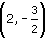.

We finish with an example that uses many of the ideas that you have met in Section 2.

Example 5

The triangle OAB has vertices at O (the origin) and at points A and B with position vectors a and b, respectively. Q is the midpoint of OB, and the point P is one-third of the way along BA from the vertex B; R is the point of intersection of the lines OP and AQ. The points P, Q and R have position vectors p, q and r, respectively.

Determine p, q and r in terms of a and b.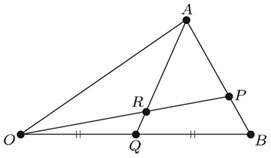The point P divides BA in the ratio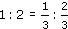. It follows from the Section Formula withthat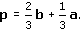Now Q is the midpoint of OB, so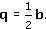Note: Here we use the fact that the position vector of O is 0 = (0, 0).

To find r, we use the fact that R is the point of intersection of OP and AQ. First, since R lies on AQ, its position vector r must be of the form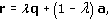for some real number λ. (r could also be written as λa + (1 − λ)q, but with a different value of λ.) Substituting from equation (2.5), we can rewrite the above formula as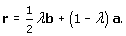The point R lies on the line OP also, so we can express its position vector r as a scalar multiple of p. Thus we can use equation (2.4) to write r in the form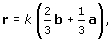for some real number k.

The expressions in equations (2.6) and (2.7) for r must be equal, so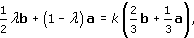which we can rewrite as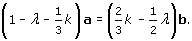We know that the vectors a and b are not parallel. Hence non-zero multiples of a and b cannot be parallel. It follows that the only way in which equation (2.8) can hold is for the coefficients of a and b in equation (2.8) to be zero: that is, we must have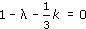and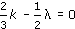. Hence the following simultaneous equations for λ and k must hold: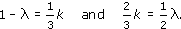Note: This is a common technique in the application of vectors to the solution of geometric problems.

From the second equation we deduce that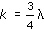. Then, substituting this value for k into the first equation, we find that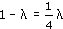; hence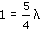, so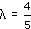.

Substituting the valuefor λ into equation (2.6), we conclude that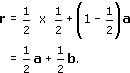Example 30

The triangle OAB has vertices at O (the origin) and at points A and B with position vectors a and b, respectively. P is the midpoint of AB, and Q is the midpoint of OA; R is the point of intersection of the lines OP and BQ. The points P, Q and R have position vectors p, q and r, respectively.

• (a) Determine p, q and r in terms of a and b.

• (b) Determine the ratio OR : RP.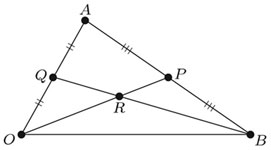• (a) Since P is the midpoint of AB, its position vector is

•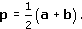• Since Q is the midpoint of OA, its position vector is

•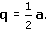• The point R lies on the two lines OP and BQ, so we can express its position vector r in two different ways. Since R lies on OP,

•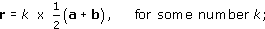• and since R lies on BQ,

•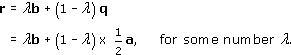• These two expressions for r must be equal; thus

•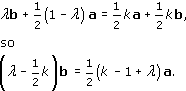• Since a and b are not parallel, their coefficients in this last equation must both be zero. This gives two equations for k and λ:

•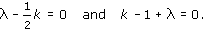• Thus k = 2λ, so 2λ − 1 + λ = 0, and hence. Hence

•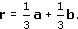• (b) The position vectors of O, R and P are 0,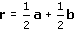and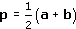, respectively. Thus

•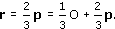• It follows from the Section Formula (with) that

•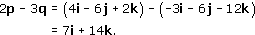• Thus R is two-thirds of the way along OP from O.

M208_1